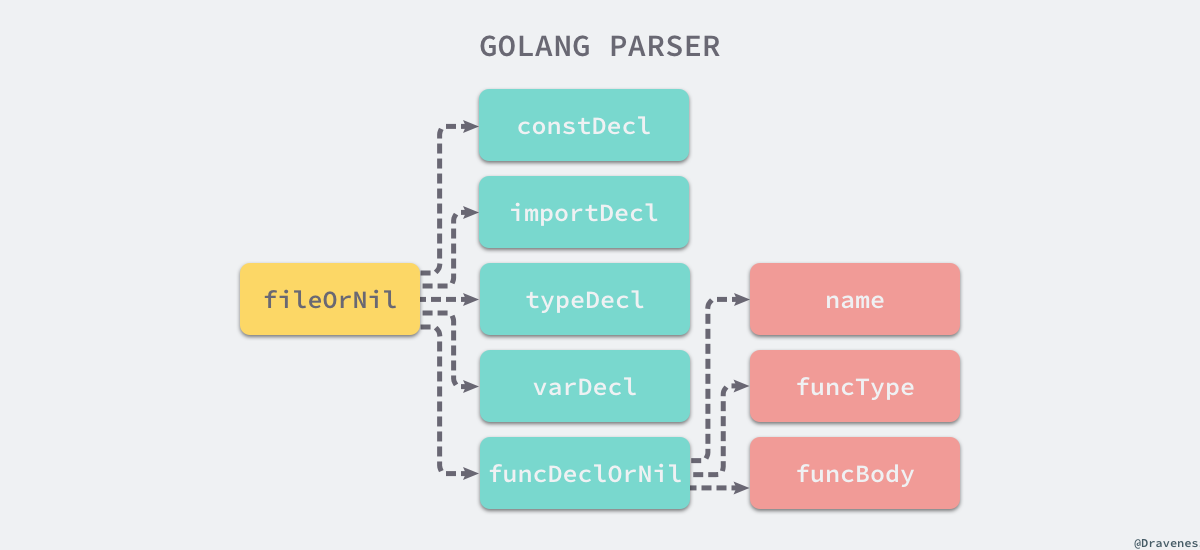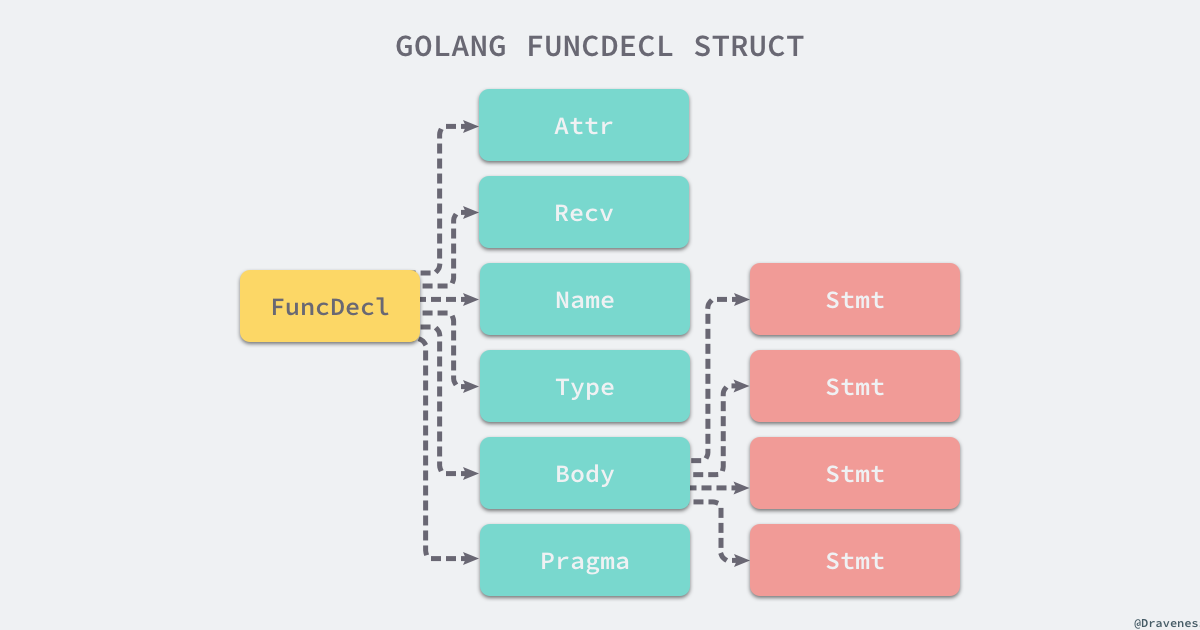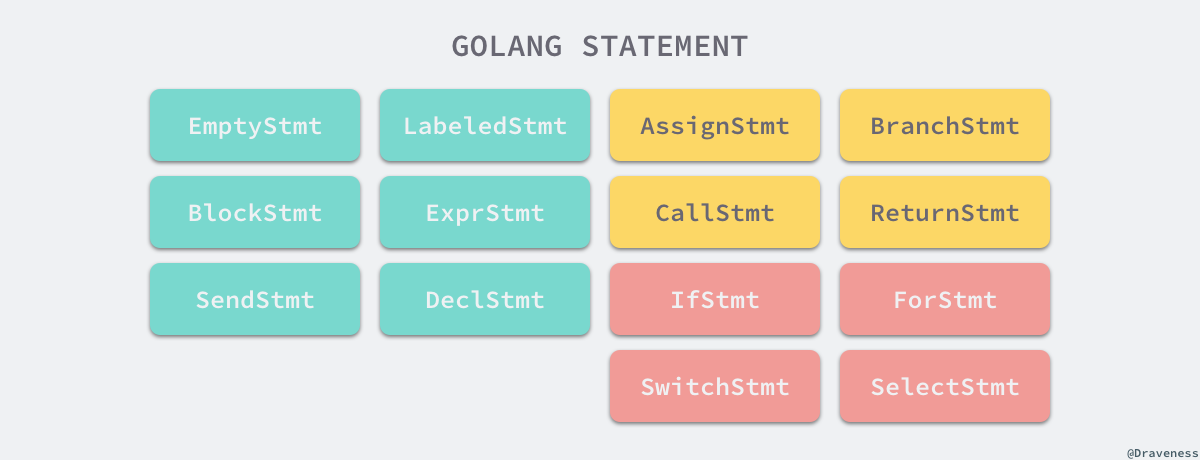1.2 词法和语法分析

词法分析

make(chan int)


lex

%{
#include <stdio.h>
%}

%%
package      printf("PACKAGE ");
import       printf("IMPORT ");
\.           printf("DOT ");
\{           printf("LBRACE ");
\}           printf("RBRACE ");
$$printf("LPAREN ");$$           printf("RPAREN ");
\"           printf("QUOTE ");
\n           printf("\n");
[0-9]+       printf("NUMBER ");
[a-zA-Z_]+   printf("IDENT ");
%%


package main

import (
"fmt"
)

func main() {
fmt.Println("Hello")
}


.l 结尾的 lex 代码并不能直接运行，我们首先需要将上述的代码『展开』成 C 语言代码，这里可以直接执行如下的命令编译并打印当前文件中的内容：

$lex simplego.l$ cat lex.yy.c
// ...
int yylex (void) {
register yy_state_type yy_current_state;
register char *yy_cp, *yy_bp;
register int yy_act;

// ...
while ( 1 ) {
yy_cp = (yy_c_buf_p);
*yy_cp = (yy_hold_char);
yy_bp = yy_cp;
yy_current_state = (yy_start);
yy_match:
do {
register YY_CHAR yy_c = yy_ec[YY_SC_TO_UI(*yy_cp)];
if ( yy_accept[yy_current_state] ) {
(yy_last_accepting_state) = yy_current_state;
(yy_last_accepting_cpos) = yy_cp;
}
while ( yy_chk[yy_base[yy_current_state] + yy_c] != yy_current_state ) {
yy_current_state = (int) yy_def[yy_current_state];
if ( yy_current_state >= 30 )
yy_c = yy_meta[(unsigned int) yy_c];
}
yy_current_state = yy_nxt[yy_base[yy_current_state] + (unsigned int) yy_c];
++yy_cp;
} while ( yy_base[yy_current_state] != 37 );

//...

do_action:
switch ( yy_act )
case 0:
*yy_cp = (yy_hold_char);
yy_cp = (yy_last_accepting_cpos);
yy_current_state = (yy_last_accepting_state);
goto yy_find_action;

case 1:
YY_RULE_SETUP
printf("PACKAGE ");
YY_BREAK
// ...
}


lex.yy.c 的前 600 行基本都是宏和函数的声明和定义，后面生成的代码大都是为 yylex 这个函数服务的，这个函数使用 有限自动机（DFA） 的程序结构来分析输入的字符流，你如果仔细看这个文件生成的代码时，会发现当前的文件中并不存在 main 函数的定义，主函数其实是在 liblex 这个库中定义的，所以在编译时其实需要添加额外的 -ll 选项：

$cc lex.yy.c -o simplego -ll$ cat main.go | ./simplego


PACKAGE  IDENT

IMPORT  LPAREN
QUOTE IDENT QUOTE
RPAREN

IDENT  IDENT LPAREN RPAREN  LBRACE
IDENT DOT IDENT LPAREN QUOTE IDENT QUOTE RPAREN
RBRACE


Go

Go 语言的词法解析是通过 cmd/compile/internal/syntax 包中的 scanner 结构实现的，这个结构体会持有当前扫描的数据源、启用的模式和当前被扫描到的 Token：

type scanner struct {
source
mode   uint
nlsemi bool

// current token, valid after calling next()
line, col uint
tok       token
lit       string
kind      LitKind
op        Operator
prec      int
}


tokens.go 文件中定义了 Go 语言中支持的全部 Token 类型，token 类型其实被定义成了正整数，在这里可以看到一些常见 Token 的类型：

const (
_    token = iota
_EOF       // EOF

// names and literals
_Name    // name
_Literal // literal

// operators and operations
_Operator // op
// ...

// delimiters
_Lparen    // (
_Lbrack    // [
// ...

// keywords
_Break       // break
// ...
_Type        // type
_Var         // var

tokenCount //
)


func (s *scanner) next() {
c := s.getr()
for c == ' ' || c == '\t' || c == '\n' || c == '\r' {
c = s.getr()
}

s.line, s.col = s.source.line0, s.source.col0

if isLetter(c) || c >= utf8.RuneSelf && s.isIdentRune(c, true) {
s.ident()
return
}

switch c {
case -1:
s.tok = _EOF

case '0', '1', '2', '3', '4', '5', '6', '7', '8', '9':
s.number(c)

// ...
}
}


scanner 每次都会通过 getr 函数获取文件中最近的未被解析的字符，然后根据当前字符的不同执行不同的 case，如果遇到了空格和换行符这些空白字符会直接跳过，如果当前字符是 0 就会执行 number 方法尝试匹配一个数字。

func (s *scanner) number(c rune) {
s.startLit()

if c != '.' {
s.kind = IntLit
for isDigit(c) {
c = s.getr()
}
}

s.ungetr()
s.nlsemi = true
s.lit = string(s.stopLit())
s.tok = _Literal
}


Go 语言的词法元素相对来说还是比较简单，使用这种巨大的 switch/case 进行词法解析也比较方便和顺手，早期的 Go 语言虽然使用 lex 这种工具来生成词法解析器，但是最后还是使用 Go 语言实现词法分析器，用自己写的词法分析器来解析自己。

语法分析

文法

• $N$ 有限个非终结符的集合；
• $\Sigma$ 有限个终结符的集合；
• $P$ 有限个生产规则的集合；
• $S$ 非终结符集合中唯一的开始符号

1. $S \rightarrow aSb$
2. $S \rightarrow ab$

SourceFile = PackageClause ";" { ImportDecl ";" } { TopLevelDecl ";" } .
PackageClause  = "package" PackageName .
PackageName    = identifier .

ImportDecl       = "import" ( ImportSpec | "(" { ImportSpec ";" } ")" ) .
ImportSpec       = [ "." | PackageName ] ImportPath .
ImportPath       = string_lit .

TopLevelDecl  = Declaration | FunctionDecl | MethodDecl .
Declaration   = ConstDecl | TypeDecl | VarDecl .


Go 语言更详细的文法可以从 Language Specification 中找到，这里不仅包含语言的文法，还包含此词法元素、内置函数等信息。

type File struct {
PkgName  *Name
DeclList []Decl
Lines    uint
node
}


ConstDecl = "const" ( ConstSpec | "(" { ConstSpec ";" } ")" ) .
ConstSpec = IdentifierList [ [ Type ] "=" ExpressionList ] .

TypeDecl  = "type" ( TypeSpec | "(" { TypeSpec ";" } ")" ) .
TypeSpec  = AliasDecl | TypeDef .
AliasDecl = identifier "=" Type .
TypeDef   = identifier Type .

VarDecl = "var" ( VarSpec | "(" { VarSpec ";" } ")" ) .
VarSpec = IdentifierList ( Type [ "=" ExpressionList ] | "=" ExpressionList ) .


FunctionDecl = "func" FunctionName Signature [ FunctionBody ] .
FunctionName = identifier .
FunctionBody = Block .

MethodDecl = "func" Receiver MethodName Signature [ FunctionBody ] .

Block = "{" StatementList "}" .
StatementList = { Statement ";" } .

Statement =
Declaration | LabeledStmt | SimpleStmt |
GoStmt | ReturnStmt | BreakStmt | ContinueStmt | GotoStmt |
FallthroughStmt | Block | IfStmt | SwitchStmt | SelectStmt | ForStmt |
DeferStmt .

SimpleStmt = EmptyStmt | ExpressionStmt | SendStmt | IncDecStmt | Assignment | ShortVarDecl .


分析方法

• 自顶向下分析：可以被看作找到当前输入流最左推导的过程，对于任意一个输入流，根据当前的输入符号，确定一个生产规则，使用生产规则右侧的符号替代相应的非终结符向下推导；
• 自底向上分析：语法分析器从输入流开始，每次都尝试重写最右侧的多个符号，这其实就是说解析器会从最简单的符号进行推导，在解析的最后合并成开始符号；

自顶向下

LL 文法就是一种使用自顶向上分析方法的文法，下面给出了一个常见的 LL 文法：

1. $S \rightarrow aS_1$
2. $S_1 \rightarrow bS_1$
3. $S_1 \rightarrow \epsilon$

1. $S$ （开始符号）
2. $aS_1$（规则 1)
3. $abS_1$（规则 2)
4. $abbS_1$（规则 2)
5. $abb$（规则 3)

自底向上

1. $S \rightarrow S_1$
2. $S_1 \rightarrow S_1b$
3. $S_1 \rightarrow a$

1. $a$（入栈）
2. $S_1$（规则 3）
3. $S_1b$（入栈）
4. $S_1$（规则 2）
5. $S_1b$（入栈）
6. $S_1$（规则 2）
7. $S$（规则 1）

Go

Go 语言的解析器就使用了 LALR(1) 的文法来解析词法分析过程中输出的 Token 序列，最右推导加向前查看构成了 Go 语言解析器的最基本原理，也是大多数编程语言的选择。

Go 语言编译过程 中介绍了编译器中的主函数，在 Main 中调用了 parseFiles 函数，这个函数主要完成了两部分工作，一部分是调用 syntax 包中的 Parse 方法对文件中的源代码进行解析，另一部分通过调用 node 函数将抽象语法树中的节点加入全局的 xtop 变量中：

func parseFiles(filenames []string) uint {
var noders []*noder

for _, filename := range filenames {
p := &noder{
basemap: make(map[*syntax.PosBase]*src.PosBase),
err:     make(chan syntax.Error),
}
noders = append(noders, p)

go func(filename string) {
base := syntax.NewFileBase(filename)

f, _ := os.Open(filename)
defer f.Close()

p.file, _ = syntax.Parse(base, f, p.error, p.pragma, syntax.CheckBranches)
}(filename)
}

var lines uint
for _, p := range noders {
p.node()
lines += p.file.Lines
}

return lines
}


func Parse(base *PosBase, src io.Reader, errh ErrorHandler, pragh PragmaHandler, mode Mode) (_ *File, first error) {
var p parser
p.init(base, src, errh, pragh, mode)
p.next()
return p.fileOrNil(), p.first
}


fileOrNil 方法其实就是对上面介绍的 Go 语言文法的实现，该方法首先会解析文件开头的 package 定义：

// SourceFile = PackageClause ";" { ImportDecl ";" } { TopLevelDecl ";" } .
func (p *parser) fileOrNil() *File {
f := new(File)
f.pos = p.pos()

if !p.got(_Package) {
p.syntaxError("package statement must be first")
return nil
}
f.PkgName = p.name()
p.want(_Semi)


    for p.got(_Import) {
f.DeclList = p.appendGroup(f.DeclList, p.importDecl)
p.want(_Semi)
}


    for p.tok != _EOF {
switch p.tok {
case _Const:
p.next()
f.DeclList = p.appendGroup(f.DeclList, p.constDecl)

case _Type:
p.next()
f.DeclList = p.appendGroup(f.DeclList, p.typeDecl)

case _Var:
p.next()
f.DeclList = p.appendGroup(f.DeclList, p.varDecl)

case _Func:
p.next()
if d := p.funcDeclOrNil(); d != nil {
f.DeclList = append(f.DeclList, d)
}
}
}

f.Lines = p.source.line

return f
}


fileOrNil 方法的最后就会返回文件最开始创建的 File 结构体，除此之外，该方法使用了非常多的子方法对输入的文件进行语法分析。

fileOrNil 与在这个方法中执行的其他子方法共同构成一颗树，根节点就是 fileOrNil 方法，子节点就是 importDeclconstDecl 等方法。SourceFile = PackageClause ";" { ImportDecl ";" } { TopLevelDecl ";" } .


辅助方法

func (p *parser) got(tok token) bool {
if p.tok == tok {
p.next()
return true
}
return false
}

func (p *parser) want(tok token) {
if !p.got(tok) {
p.syntaxError("expecting " + tokstring(tok))
}
}


got 其实只是用于快速判断一些语句中的关键字，如果当前解析器中的 Token 是传入的 Token 就会直接跳过该 Token 并返回 true；而 want 就是对 got 的简单封装了，如果当前的 Token 不是我们期望的，就会立刻返回语法错误并结束这次编译。

var (
a int
b int
)


func (p *parser) appendGroup(list []Decl, f func(*Group) Decl) []Decl {
if p.tok == _Lparen {
g := new(Group)
p.list(_Lparen, _Semi, _Rparen, func() bool {
list = append(list, f(g))
return false
})
} else {
list = append(list, f(nil))
}

return list
}


appendGroup 方法会调用传入的 f 方法对输入流进行匹配并将匹配的结果追加到另一个参数 File 结构体中的 DeclList 数组中，importconstvartypefunc 声明语句都是调用 appendGroup 方法进行解析的。

节点

type File struct {
PkgName  *Name
DeclList []Decl
Lines    uint
node
}



type (
Decl interface {
Node
}

FuncDecl struct {
Attr   map[string]bool
Recv   *Field
Name   *Name
Type   *FuncType
Body   *BlockStmt
Pragma Pragma
decl
}
}